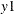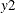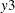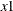The VARMAX Procedure

CAUSAL Statement

• CAUSAL GROUP1=(variables) GROUP2=(variables);

A CAUSAL statement prints the Granger causality test by fitting the VAR(p) model by using all variables defined in GROUP1 and GROUP2. Any number of CAUSAL statements can be specified. The CAUSAL statement proceeds with the MODEL statement and uses the variables and the autoregressive order, p, specified in the MODEL statement. Variables in the GROUP1= and GROUP2= options should be defined in the MODEL statement. If the P=0 option is specified in the MODEL statement, the CAUSAL statement is not applicable.

The null hypothesis of the Granger causality test is that GROUP1 is influenced only by itself, and not by GROUP2. If the hypothesis test fails to reject the null, then the variables listed in GROUP1 might be considered as independent variables.

See the section VAR and VARX Modeling for details.

The following is an example of the CAUSAL statement. You specify the CAUSAL statement with the GROUP1= and GROUP2= options.

```   proc varmax data=one;
model y1-y3 = x1 / p=1;
causal group1=(x1) group2=(y1-y3);
causal group1=(y2) group2=(y1 y3);
run;
```

The first CAUSAL statement fits the VAR(1) model by using the variables,,, andand tests the null hypothesis thatcauses the other variables,,, and, but the other variables do not cause. The second CAUSAL statement fits the VAR(1) model by using the variables,, andand tests the null hypothesis thatcauses the other variables,and, but the other variables do not cause.